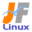Linux Kernel 2.6 Documentation: /usr/src/linux/Documentation/usb/uhci.txt

# usb/uhci.txt

\$B?7\$7\$\$(B UHCI \$B%I%i%\$%P\$N(B \$B;EMM\$HFbIt(B [\$B%W%l%\$%s%F%-%9%HHG(B]
• \$B86Cx:n
• \$BK]Lu
• \$B%P!<%8%g%s(B: 2.6.14
• \$BK]LuF|;~(B: 2005/11/13
```

==================================
\$B\$3\$l\$O!"(B
linux-2.6.14/Documentation/usb/uhci.txt \$B\$NOBLu(B
\$B\$G\$9!#(B
\$BK]LuCDBN!'(B JF \$B%W%m%8%'%/%H(B < http://www.linux.or.jp/JF/ >
\$B99?7F|(B \$B!'(B 2005/11/13
\$B86Cx:nl=j\$K\$"\$j\$^\$9!#(B

http://hotswap.in.tum.de/usb

1. General issues

1. \$B0lHLE*\$JLdBj(B

1.1 Why a new UHCI driver, we already have one?!?

1.1 \$B\$9\$G\$K%I%i%\$%P\$,\$"\$k\$N\$K!"\$J\$Aw(B (ISO) \$B\$rF0\$+\$9\$?\$a\$N(B
(\$B8=:_\$bB3\$1\$i\$l\$F\$\$\$k(B) \$BEXNO\$K\$h\$j!"\$^\$9\$^\$9:.Mp\$7\$F\$\$\$^\$9!#(B
\$B0lJ}!"0BDj\$7\$?(B ISO \$BE>Aw\$O\$^\$9\$^\$9I,MW(B
(\$BFC\$K!"(BTS(Thomas Sailer \$B;a(B) \$B\$,I,MW\$J(B USB \$B%*!<%G%#%*\$H!"(BGA(Georg Acher \$B;a(B) \$B\$H!"(B
DF(Deti Fliegl \$B;a(B) \$B\$N:n\$C\$?(B DAB-USB \$B%l%7!<%P\$N\$?\$a\$K(B) \$B\$H\$J\$C\$F\$\$\$^\$9!#\$3\$N>uBV(B
\$B\$K!"(B(\$B;dC#\$N0U8+\$H\$7\$F(B) \$B>/!9GMQ\$5\$l!"(B
\$BN>J}\$N%I%i%\$%P\$,!"6&DL\$N(B API \$B\$r6&M-\$9\$k\$h\$&\$K\$J\$k\$3\$H\$rK>\$s\$G\$\$\$^\$9!#(B

1.2. \$BMxE@\$H7gE@(B

+ All USB transfer types work now!
+ Asynchronous operation
+ Simple, but powerful interface (only two calls for start and cancel)
+ Easy migration to the new API, simplified by a compatibility API
+ Simple usage of ISO transfers
+ ISO transfers allow variable length for each frame and striping
+ No CPU dependent and non-portable atomic memory access, no asm()-inlines
+ Tested on x86 and Alpha

+ \$B8=:_(B \$B\$9\$Y\$F\$NAw(B \$B\$,F0:n\$7\$^\$9!*(B
+ \$BHsF14|=hM}(B
+ \$BC1=c\$G6/NO\$J%\$%s%?%U%'!<%9(B (\$B3+;O\$HC\$N\$?\$a!"(B2\$B2s8F\$S=P\$9\$@\$1\$GNI\$\$(B)
+ \$B?7\$7\$\$(B API \$B\$X\$N0\9T\$,4JC1(B (\$B8_49(B API \$B\$K\$h\$k4JN,2=(B)
+ \$BC1=c\$J(B ISO \$BE>Aw\$N;HMQK!(B
+ \$B%j%/%(%9%H\$N<+F0O"7k(B
+ ISO \$BE>Aw\$O!"\$=\$l\$>\$l\$N%U%l!<%`\$H%9%H%i%\$%T%s%0\$r2DJQD9\$K\$G\$-\$^\$9!#(B
+ CPU \$B\$K0MB8\$7\$J\$\$!"0\?"\$G\$-\$J\$\$%"%H%_%C%/\$J%a%b%j%"%/%;%9\$r;H\$o\$J\$\$!"(B
\$B%\$%s%i%\$%s(B asm() \$B\$r;H\$o\$J\$\$!#(B
+ x86 \$B\$H!"(BAlpha \$B\$G%F%9%H\$7\$F\$\$\$^\$9!#(B

- Rewriting for ISO transfers needed

- ISO \$BE>Aw\$r;H\$&\$K\$O!"=q\$-49\$(\$,I,MW\$G\$9(B

1.3. Is there some compatibility to the old API?

1.3. \$B8E\$\$(B API \$B\$H\$N8_49@-\$O\$"\$j\$^\$9\$+!)(B

Yes, but only for control, bulk and interrupt transfers. We've implemented
some wrapper calls for these transfer types. The usbcore works fine with
these wrappers. For ISO there's no compatibility, because the old ISO-API
and its semantics were unnecessary complicated in our opinion.

\$B8_49@-\$O\$"\$j\$^\$9\$,!"%3%s%H%m!<%kE>Aw\$H%P%k%/E>Aw\$H%\$%s%?%i%W%HE>Aw\$@\$1\$G\$9!#(B
\$B\$3\$l\$iE>Aw%?%\$%W\$r8F\$S=P\$9!"\$\$\$/\$D\$+\$N%i%C%Q\$re=R\$7\$?\$h\$&\$K!"(BCTRL \$B\$H(B BULK \$B\$,\$=\$l\$>\$l!"%i%C%Q\$rMQ\$\$\$?>uBV\$G\$b\$&\$^\$/F0\$\$\$F\$\$(B
\$B\$^\$9\$N\$G!"8E\$\$%3!<%I\$OJQ99\$K5\$\$,\$D\$+\$J\$\$\$G\$7\$g\$&!#(B
Thomas \$BC4Ev4XO"\$G\$O!"(BISO \$BE>Aw\$O!"8=:_(B USB \$B%*!<%G%#%*\$G0BDjF0:n\$7\$F\$\$\$^\$9!#(B
INT \$BE>Aw(B (\$B%^%&%9%I%i%\$%P\$J\$I(B) \$B\$b!"\$&\$^\$/F0\$-\$^\$9!#(B

1.5. Are there any bugs?

1.5. \$B\$I\$s\$J%P%0\$,\$"\$j\$^\$9\$+!)(B

No ;-)
Hm...
Well, of course this implementation needs extensive testing on all available
hardware, but we believe that any fixes shouldn't harm the overall concept.

\$B\$"\$j\$^\$;\$s(B ;-)
\$B\$&!<\$s(B...
\$B\$=\$&\$G\$9\$M!"\$b\$A\$m\$s!"\$3\$N\$N(B USB \$B%G%P%\$%9%I%i%\$%P\$O!"%"%\$%=%/%m%J%9E>Aw\$b\$7\$/\$O%\$%s%?%i%W%HE>Aw(B
\$B\$r;H\$&\$J\$i!"(BURB \$B2=\$7\$J\$1\$l\$P\$\$\$1\$^\$;\$s!#8=:_\$NAw\$N\$?\$a\$N8xJ?\$JBT\$A9TNs\$G\$9!#\$3\$l\$K4X\$7\$F\$O!"\$9\$G\$K(B
\$B9=C[\$5\$l\$?(B TD \$B%A%'%\$%s(B (\$B?<\$5\$+\$iI}\$X\$NJQ99\$rpJs8;\$O!"(Buhci.c \$B\$@\$1\$G\$9!*(B

2.1. Descriptor structure (QHs and TDs)

2.1. \$B%G%#%9%/%j%W%?\$N9=B\$(B (QH \$B\$H(B TD)

During initialization, the following skeleton is allocated in init_skel:

\$B=i4|2=Cf!"0J2<\$N9|AH\$_\$,!"(Binit_skel \$BFb\$KG[CV\$5\$l\$^\$9!#(B

framespecific           |           common chain

\$B%U%l!<%`L@:Y(B            \$B!C(B           \$B6&DL%A%'%\$%s(B

framelist[]
[  0 ]-----> TD --> TD -------\
[  1 ]-----> TD --> TD --------> TD ----> QH -------> QH -------> QH ---> NULL
...        TD --> TD -------/
-----> TD --> TD ------/

^^     ^^           ^^       ^^          ^^          ^^
1024 TDs for   7 TDs for    1 TD for   Start of    Start of    End Chain
ISO  INT (2-128ms) 1ms-INT    CTRL Chain  BULK Chain

ISO \$BMQ(B TD     INT (2-128ms) 1ms-INT \$BMQ(B CTRL        BULK        \$B=*N;%A%'%\$%s(B
1024\$B8D(B       \$BMQ(B TD 7\$B8D(B     TD 1\$B8D(B    \$B%A%'%\$%s(B    \$B%A%'%\$%s(B
\$B\$N;O\$^\$j(B    \$B\$N;O\$^\$j(B

For each CTRL or BULK transfer a new QH is allocated and the containing data
transfers are appended as (vertical) TDs. After building the whole QH with its
dangling TDs, the QH is inserted before the BULK Chain QH (for CTRL) or
before the End Chain QH (for BULK). Since only the QH->next pointers are
affected, no atomic memory operation is required. The three QHs in the
common chain are never equipped with TDs!

CTRL \$BE>Aw\$d(B BULK \$BE>Aw\$=\$l\$>\$l\$K\$O!"?7\$7\$\$(B QH \$B\$,3d\$jEv\$F\$i\$l!"E>Aw\$5\$l\$k%G!<%?(B
\$B\$O!"(BTD \$B\$H\$7\$F!"(B(\$B?bD>J}8~\$K(B) \$BDI2C\$5\$l\$^\$9!#(BTD \$B\$,IU\$1\$i\$l\$?(B QH \$BA4BN\$r9=C[\$7\$?\$"\$H!"(B
QH \$B\$O!"(BBULK \$B%A%'%\$%s\$N(B QH \$B\$NA0(B (CTRL \$BE>Aw(B) \$B!"\$^\$?\$O!"(BEnd \$B%A%'%\$%s\$N(B QH \$B\$NA0(B
(BULK \$BE>Aw(B) \$B\$KA^F~\$5\$l\$^\$9!#\$3\$N\$H\$-(B QH->next \$B%]%\$%s%?\$@\$1\$,1F6A\$r\$l\$N%U%l!<%`\$N\$?\$a\$N(B TD \$B\$O!"4uK>\$9\$k%U%l!<%`\$N(B
\$BE,@Z\$J(B ISO/INT-TD \$B%A%'%\$%s\$KC1\$KA^F~\$5\$l\$k\$@\$1\$G\$9!#(B7\$B\$D\$N(B \$B9|AH\$_\$N(B INT-TD \$B\$O!"(B
\$B8E\$\$(B UHCI \$B%I%i%\$%P\$HF1MM\$K(B 1024 \$B%U%l!<%`\$KJ,;6\$5\$l\$^\$9!#(B

For CTRL/BULK/ISO, the last TD in the transfer has the IOC-bit set. For INT,
every TD (there is only one...) has the IOC-bit set.

CTRL/BULK/ISO \$B\$G!"E>AwCf!":G8e\$N(B TD \$B\$O!"(BIOC \$B%S%C%H\$,%;%C%H\$5\$l\$F\$\$\$^\$9!#(B
INT \$B\$G!"\$9\$Y\$F\$N(B TD (\$B\$H\$\$\$C\$F\$b\$R\$H\$D\$G\$9\$,(B...) \$B\$O!"(BIOC \$B%S%C%H\$,%;%C%H\$5\$l\$F\$\$\$^\$9!#(B

Besides the data for the UHCI controller (2 or 4 32bit words), the descriptors
are double-linked through the .vertical and .horizontal elements in the
SW data of the descriptor (using the double-linked list structures and
operations), but SW-linking occurs only in closed domains, i.e. for each of
the 1024 ISO-chains and the 8 INT-chains there is a closed cycle. This
simplifies all insertions and unlinking operations and avoids costly
bus_to_virt()-calls.

UHCI \$B%3%s%H%m!<%i\$N\$?\$a\$N%G!<%?(B (2 \$B\$^\$?\$O(B 4 32\$B%S%C%H%o!<%I(B) \$B\$K2C\$(\$F!"(B
\$B%G%#%9%/%j%W%?\$O!"%G%#%9%/%j%W%?(B (\$B%@%V%k%j%s%/%j%9%H\$N9=B\$BN\$H=hM}\$r;H\$&(B) \$B\$N(B
SW \$B%G!<%?Fb\$N(B .vertical \$B\$H!"(B.horizontal \$BMWAG\$K\$h\$j!"%@%V%k%j%s%/\$5\$l\$F\$\$\$^\$9\$,!"(B
SW \$B%G!<%?\$K\$h\$k%j%s%/\$OJD\$8\$?NN0hFb\$@\$1\$GH/@8\$7\$^\$9!#\$9\$J\$o\$A!"(B1024 ISO \$B%A%'%\$(B
\$B%s\$H!"(B8 INT \$B%A%'%\$%s\$G!"3F!9JD\$8\$?NX\$K\$J\$C\$F\$\$\$^\$9!#\$3\$l\$O!"\$9\$Y\$F\$NA^F~\$H%"%s(B
\$B%j%s%/=hM}\$r4JN,2=\$7!"9b2A\$J(B bus_to_virt() \$B\$N8F\$S=P\$7\$r2sHr\$7\$^\$9!#(B

2.2. URB structure and linking to QH/TDs

2.2. URB \$B9=B\$BN\$H(B QH/TD \$B\$X\$NO"7k(B

During assembly of the QH and TDs of the requested action, these descriptors
are stored in urb->urb_list, so the allocated QH/TD descriptors are bound to
this URB.
If the assembly was successful and the descriptors were added to the HW chain,
the corresponding URB is inserted into a global URB list for this controller.
This list stores all pending URBs.

\$BMW5a\$5\$l\$?F0:n\$KBP\$7\$F(B QH \$B\$H(B TD \$B\$NAH\$_N)\$FCf!"\$3\$l\$i%G%#%9%/%j%W%?\$O!"(B
urb->urb_list \$B\$KJ]B8\$5\$l\$k\$N\$G!"3d\$jEv\$F\$i\$l\$?(B QH/TD \$B%G%#%9%/%j%W%?\$O!"\$3\$N(B
URB \$B\$K7k\$S\$D\$1\$i\$l\$^\$9!#AH\$_N)\$F\$K@.8y\$7!"%G%#%9%/%j%W%?\$,(B HW \$B%A%'%\$%s\$KDI2C\$5(B
\$B\$l\$?\$J\$i!"BP1~\$9\$k(B URB \$B\$O!"\$3\$N%3%s%H%m!<%iMQ\$N!"%0%m!<%P%k(B URB \$B%j%9%H\$KDI2C\$5(B
\$B\$l\$^\$9!#\$3\$N%j%9%H\$K\$O!"\$9\$Y\$F\$N%Z%s%G%#%s%0\$G\$"\$k(B URB \$B\$,5-21\$5\$l\$F\$\$\$^\$9!#(B

2.3. Interrupt processing

2.3. \$B3d\$j9~\$_=hM}(B

Since UHCI provides no means to directly detect completed transactions, the
following is done in each UHCI interrupt (uhci_interrupt()):

UHCI \$B\$O!"=*N;\$7\$?%H%i%s%6%/%7%g%s\$rD>@\8!CN\$9\$k\$l\$N(B UHCI \$B3d\$j9~\$_(B (uhci_interrupt()) \$B\$KBP\$7\$F0J2<\$N=hM}\$,9T\$o\$l\$^\$9(B:

For each URB in the pending queue (process_urb()), the ACTIVE-flag of the
associated TDs are processed (depending on the transfer type
process_{transfer|interrupt|iso}()). If the TDs are not active anymore,
they indicate the completion of the transaction and the status is calculated.
Inactive QH/TDs are removed from the HW chain (since the host controller
already removed the TDs from the QH, no atomic access is needed) and
eventually the URB is marked as completed (OK or errors) and removed from the
pending queue. Then the next linked URB is submitted. After (or immediately
before) that, the completion handler is called.

\$B%Z%s%G%#%s%0%-%e!<(B (process_urb()) \$B\$K\$"\$k!"\$=\$l\$>\$l\$N(B URB \$B\$G!"4XO"\$7\$?(B TD \$B\$N(B
ACTIVE \$B%U%i%0\$,=hM}\$5\$l\$^\$9(B (\$BE>Aw%?%\$%W(B process_{transfer|interrupt|iso}()
\$B\$K=>\$C\$F(B)\$B!#\$=\$N(B TD \$B\$,F0:nCf\$G\$J\$\$\$J\$i!"\$=\$l\$O%H%i%s%6%/%7%g%s\$N40N;\$r<(\$7\$F\$*\$j!"(B
\$B=*N;%9%F!<%?%9\$r7W;;\$7\$^\$9!#F0:nCf\$G\$J\$\$(B QH/TD \$B\$O!"(BHW \$B%A%'%\$%s\$+\$i:o=|\$7(B (\$B%[%9(B
\$B%H%3%s%H%m!<%i\$O\$9\$G\$K(B QH \$B\$+\$i(B TD \$B\$r:o=|:Q\$_\$J\$N\$G!"%"%H%_%C%/\$J%"%/%;%9\$OI,MW(B
\$B\$"\$j\$^\$;\$s(B)\$B!":G=*E*\$K!"\$=\$N(B URB \$B\$O!"40N;\$7\$?\$H\$7\$F0u(B (OK \$B\$^\$?\$O!"(Berror) \$B\$rIU\$1(B
\$B\$i\$l!"BT\$A9TNs\$+\$iA0(B) \$B!"40N;%O%s%I%i\$,8F\$S=P\$5\$l\$^\$9!#(B